login  home  contents  what's new  discussion  bug reports help  links  subscribe  changes  refresh  edit

Author: Martin Rubey

A more thorough discussion of this package can be found at http://arxiv.org/abs/math.CO/0702086

fricas
)set output tex off

fricas
)set output algebra on

If you find a bug please report in FriCAS BugTracker.

Finally, please feel free to try this package in the SandBox! If you would like to use this program at your own computer, you need to install FriCAS.

If you find the package useful, please let me know!

Abstract We present a software package that guesses formulas for sequences of, for example, rational numbers or rational functions, given the first few terms. Thereby we extend and complement Christian Krattenthaler's program Rate and the relevant parts of Bruno Salvy and Paul Zimmermann's GFUN.

This research was partially supported by the Austrian Science Foundation FWF, grant S8302-MAT.

## Introduction

For some a brain-teaser, for others one step in proving their next theorem: given the first few terms of a sequence of, say, integers, what is the next term, what is the general formula? Of course, no unique solution exists, however, by Occam's razor, we will prefer a "simple" formula over a more "complicated" one.

Some sequences are very easy to "guess", like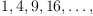(1)
or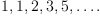(2)

Others are a little harder, for example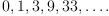(3)

Of course, at times we might want to guess a formula for a sequence of polynomials, too: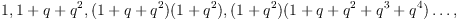(4)
or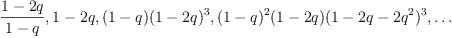(5)

Fortunately, with the right tool, it is a matter of a moment to figure out formulas for all of these sequences. In this article we describe a computer program that encompasses well known techniques and adds new ideas that we hope to be very effective. In particular, we generalize both Christian Krattenthaler's program Rate, and the guessing functions present in GFUN written by Bruno Salvy and Paul Zimmermann. With a little manual aid, we can guess multivariate formulas as well, along the lines of Doron Zeilberger's programs GuessRat and GuessHolo.

We would also like to mention The online encyclopedia of integer sequences of Neil Sloane. There, you can enter a sequence of integers and chances are good that the website will respond with one or more likely matches. However, the approach taken is quite different from ours: the encyclopedia keeps a list of currently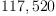sequences, entered more or less manually, and it compares the given sequence with each one of those. Besides that, it tries some simple transformations on the given sequence to find a match. Furthermore it tries some simple programs we will describe below to find a formula, although with a time limit, i.e., it gives up when too much time has elapsed.

Thus, the two approaches complement each other: For example, there are sequences where no simple formula is likely to exist, and which can thus be found only in the encyclopedia. On the other hand, there are many sequences that have not yet found their way into the encyclopedia, but can be guessed in a few minutes by your computer.

On the historical side, we remark that already in 1966 Paul W. Abrahams implemented a program to identify sequences given their first few terms...

## Safety and Speed

A formula for Sequence (1) is almost trivial to guess: it seems obvious that it is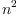. More generally, if we believe that the sequence in question is generated by a polynomial, we can simply apply interpolation. However, how can we know that a polynomial formula is appropriate? The answer is quite simple: we use all but the last few terms of the sequence to derive the formula. After this, the last terms are compared with the values predicted by the polynomial. If they coincide, we can be confident that the guessed formula is correct. We call the number of terms used for checking the formula the safety of the result.

Apart from safety, the main problem we have to solve is about efficiency. For example, maybe we would like to test whether the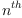term of the sequence is given by a formula of the form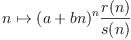(6)
for some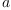and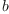and polynomials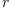and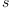. Of course, we could set up an appropriate system of polynomial equations. However, it would usually take a very long time to solve this system.

Thus, we need to find efficient algorithms that test for large classes of formulas. Obviously, such algorithms exist for interpolation and Pade approximation. For the present package, we implemented an efficient algorithm for a far reaching generalization of interpolation, proposed by Bernhard Beckermann and George Labahn, see FractionFreeFastGaussianElimination. Furthermore, we show that there is also a way to guess sequences generated by Formula (6).

Using these algorithms our package clearly outperforms both Rate and GFUN, in terms of speed as well as in the range of formulas that can be guessed.

In the following section we outline the capabilities of our package. In the Section therafter we describe the most important options that modify the behaviour of the functions.

## Function Classes Suitable for Guessing

In this section we briefly present the function classes which are covered by our package. Throughout this section,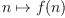is the function we would like to guess, and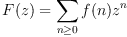is its generating function. The values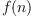are supposed to be elements of some field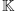, usually the field of rationals or rational functions. We alert the reader that the first value in the given sequence always corresponds to the value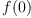.

### Guessing• guessRec finds recurrences of the form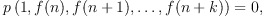(7)
where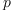is a polynomial with coefficients in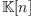. For example,
fricas
guessRec([1,1,0,1,- 1,2,- 1,5,- 4,29,- 13,854,- 685])
2
(1)  [[f(n): f(n + 2) + f(n + 1) - f(n)  = 0, f(0) = 1, f(1) = 1]]
Type: List(Expression(Integer))

Note that, at least in the current implementation, we do not exclude solutions that do not determine the function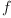completely. For example, given a list containing only zeros and ones, one result will be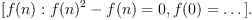• guessPRec only looks for recurrences with linear, i.e., it recognizes P-recursive sequences. As an example,
fricas
guessPRec([0, 1, 0, -1/6, 0, 1/120, 0, -1/5040, 0, 1/362880, 0, -1/39916800])
(2)  []
Type: List(Expression(Integer))
• guessRat finds rational functions. For the sequence given in Equation (1), we findas likely solution.
• guessExpRat finds rational functions with an Abelian term, i.e.,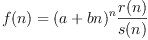whereandare polynomials.
fricas
guessExpRat([0,3,32,375,5184,84035])
n
(3)  [n (n + 2) ]
Type: List(Expression(Integer))

Concerning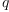-analogues, guessRec(q) finds recurrences of the form (7), whereis a polynomial with coefficients in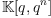. Similarly, we provide-analogues for guessPRec and guessRat. Finally, guessExpRat(q) recognizes functions of the form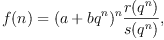andbeing in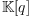andandpolynomials with coefficients in. This includes, for example, Nicholas Loehr's-analogue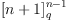of Cayley's formula, where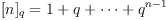.

For Sequence (5), we enter

fricas
guessExpRat(q)([(1-2*q)/(1-q),1-2*q,(1-q)*(1-2*q)^3,(1-q)^2*(1-2*q)*(1-2*q-2*q^2)^3], [])
n           n
(2 q - 1)(2 q  - 3 q + 1)
(4)  [--------------------------]
q - 1
Type: List(Expression(Integer))

## Guessing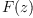• guessADE finds an algebraic differential equation for, i.e., an equation of the form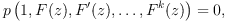(8)
whereis a polynomial with coefficients in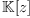. A typical example is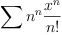:
fricas
guessADE([1,1,2,9/2,32/3,625/24,324/5,117649/720,131072/315])
(5)  []
Type: List(Expression(Integer))
• guessHolo only looks for equations of the form (11) with linear, that is, it recognizes holonomic or differentially-finite functions. It is well known that the class of holonomic functions coincides with the class of functions having P-recursive Taylor coefficients. However, the number of terms necessary to find the differential equation often differs greatly from the number of terms necessary to find the recurrence. Returning to the example given for guessPRec, we find that already the first 6 terms are sufficient to guess a generating function:
fricas
guessHolo([0,1,0,-1/6,0,1/120])
3
n        ,,                           x       4
(6)  [[[x ]f(x): f  (x) + f(x) = 0, f(x) = x - -- + O(x )]]
6
Type: List(Expression(Integer))

Moreover, now we immediately recognise the coefficients as being those of the sine function. guessHolo is also the function provided by GFUN. Here is a comparison of average running times in seconds over several runs on the same machine for a list of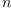elements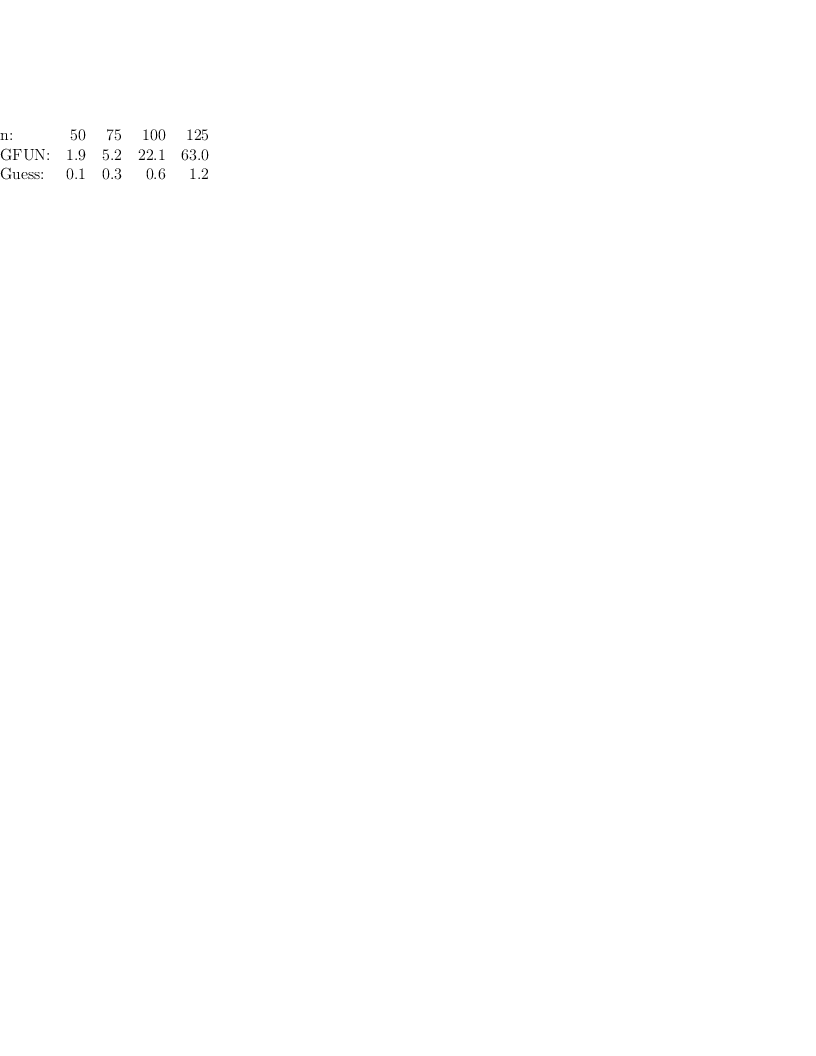• guessAlg looks for an algebraic equation satisfied by, i.e., an equation of the form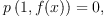the prime example being given by the Catalan numbers
fricas
first guessAlg [1,1,2,5,14,42]
n             2                                   2      3      4
(7)  [[x ]f(x): x f(x)  - f(x) + 1 = 0, f(x) = 1 + x + 2 x  + 5 x  + O(x )]
Type: Expression(Integer)
• guessPade recognises rational generating functions. For the Fibonacci sequence given in Equation (2), we find as likely solution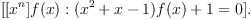We provide-analogues, replacing differentiation with-dilation: guessADE(q) finds differential equations of the form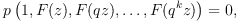(9)
whereis a polynomial with coefficients in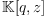. Similarly, there are-analogues for guessHolo, guessAlg, and guessPade.

To guess a formula for Sequence (4), we enter

fricas
guessRat(q)([1,1+q+q^2,(1+q+q^2)*(1+q^2),(1+q^2)*(1+q+q^2+q^3+q^4)], [])
3 2 n       2      n
q q    + (- q  - q)q  + 1
(8)  [-------------------------]
3    2
q  - q  - q + 1
Type: List(Expression(Integer))

## Operators

The main observation made by Christian Krattenthaler in designing his program Rate is the following: it occurs frequently that although a sequence of numbers is not generated by a rational function, the sequence of successive quotients is.

We slightly extend upon this idea, and apply recursively one or both of the two following operators:

• guessSum -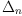the differencing operator, transforminginto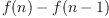.
• guessProduct -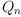the operator that transformsinto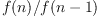.

For example, to guess a formula for Sequence (3), we enter

fricas
guess([0, 1, 3, 9, 33], [guessRat], [guessSum, guessProduct])
s  - 1
n - 1    5
--+    ++-++
(9)  [ >       | |    p  + 2]
--+     | |     4
s  = 0  p  = 0
5       4
Type: List(Expression(Integer))

The second argument to guess indicates which of the functions of the previous section to apply to each of the generated sequence, while the third argument indicates which operators to use to generate new sequences.

In the case where only the operatoris applied, our package is directly comparable to Rate. In this case the standard example is the number of alternating sign matrices

fricas
guess([1, 1, 2, 7, 42, 429, 7436, 218348], [guessRat], [guessProduct])
p  - 1       2
n - 1    8      27 p   + 54 p  + 24
++-++   ++-++       7        7
(10)  [ | |     | |    -------------------]
| |     | |         2
p  = 0  p  = 0  16 p   + 32 p  + 12
8       7          7        7
Type: List(Expression(Integer))

Here are the average running times in seconds for our package and Rate over several runs on the same machine for a list ofelements:: & 14 & 15 & 16 & 17 & 18\ Rate: & 1.0 & 3.3 & 29.7 & 44.9 & 398\ Guess: & 0.9 & 2.3 & 6.6 & 22.4 & 74 " title="{lrrrrrrrrrr} $n$: & 14 & 15 & 16 & 17 & 18\ Rate: & 1.0 & 3.3 & 29.7 & 44.9 & 398\ Guess: & 0.9 & 2.3 & 6.6 & 22.4 & 74 " class="equation" src="images/2257039485981635045-16.0px.png" align="bottom" Style="vertical-align:text-bottom" width="204" height="52"/>

## Options

To give you the maximum flexibility in guessing a formula for your favourite sequence, we provide options that modify the behaviour of the functions as described in Section~\ref{sec:function-classes}. The options are appended, separated by commas, to the guessing function in the form \spad{option==value}. See below for some examples.

• debug specifies whether informations about progress should be reported.
• safety specifies, as explained at the beginning of Section 2, the number of values reserved for testing any solutions found. The default setting is 1.

Experiments seem to indicate that for guessADE higher settings are appropriate than for guessRat. I.e., if a rational function interpolates the given list of terms, where the final term is used for testing, we can be pretty sure that the formula found is correct. By contrast, we recommend setting safety to 3 or 4 when using guessADE. For all algorithms except guessExpRat we recommend to omit trailing zeros.

• one specifies whether the guessing function should return as soon as at least one solution is found. By default, this option is set to true.
• maxDegree specifies the maximum degree of the coefficient polynomials in an algebraic differential equation or a recursion with polynomial coefficients. For rational functions with an exponential term, maxDegree bounds the degree of the denominator polynomial. This option is especially interesting if trying rather long sequences where it is unclear whether a solution will be found or not. Setting maxDegree to -1, which is the default, specifies that the maximum degree can be arbitrary.
• allDegrees specifies whether all possibilities of the degree vector - taking into account maxDegree - should be tried. The default is true for guessPade and guessRat and false for all other functions.
• homogeneous specifies whether the search space should be restricted to homogeneous algebraic differential equations or homogeneous recurrences. By default, it is set to false.
• maxDerivative - maxShift specify the maximum derivative in an algebraic differential equation, or, in a recurrence relation, the maximum shift. Setting the option to -1 specifies that the maximum derivative - the maximum shift - may be arbitrary.
• maxPower specifies the maximum total degree in an algebraic differential equation or recurrence: for example, the degree of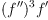is 4. Setting the option to -1 specifies that the maximum total degree may be arbitrary. For example,
fricas
l := [1, 1, 1, 1, 2, 3, 7, 23, 59, 314, 1529, 8209, 83313, 620297, 7869898, 126742987, 1687054711, 47301104551, 1123424582771, 32606721084786, 1662315215971057];
Type: List(PositiveInteger?)
fricas
guessRec(l, maxPower==2)
(12)
[
2
[f(n): - f(n)f(n + 4) + f(n + 1)f(n + 3) + f(n + 2)  = 0, f(0) = 1,
f(1) = 1, f(2) = 1, f(3) = 1]
]
Type: List(Expression(Integer))

returns the Somos-4 recurrence, whereas without limiting the power to 2, we need the first 33 values, and instead of roughly one second half a minute of computing time.

• maxLevel specifies how many levels of recursion are tried when applying operators. Note that, applying either of the two operators results in a sequence which is by one shorter than the original sequence. Therefore, in case both guessSum and guessProduct are specified, the number of times a guessing algorithm from the given list of functions is applied is roughly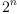, where $n$ is the number of terms in the given sequence. Thus, especially when the list of terms is long, it is important to set maxLevel to a low value.

Still, the default value is -1, which means that the number of levels is only restricted by the number of terms given in the sequence.

• indexName, variableName, functionName specify symbols to be used for the output. The defaults are n, x and f respectively.

## A note on the output

The output of any function described in Section 3 is a list of formulae which seem to fit, along with an integer that states from which term on the formula is correct. The latter is necessary, because rational interpolation features sometimes unattainable points, as the following example shows:

fricas
guessRat([3, 4, 7/2, 18/5, 11/3, 26/7])
2                   2
(13)  [[f(n): (- n  - n + 2)f(n) + 4 n  + 2 n - 6 = 0]]
Type: List(Expression(Integer))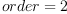indicates that the first two terms of the sequence might not coincide with the value predicted by the returned function. A similar situation occurs, if the function generating the sequence has a singular point at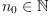, where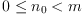and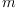is the number of given values. We would like to stress that this is rather a feature than a bug: most terms will be correct, just as in the example above, where the value at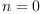is indeed 3.

Fricas Version --Bill Page, Wed, 14 Oct 2009 10:33:47 -0700 reply
fricas
)version
Value = "FriCAS 1.3.5 compiled at Thu Feb 21 18:22:14 UTC 2019"

 Subject:   Be Bold !! ( 14 subscribers )